Home | ARTS | Financial Management | Cost of Equity Capital - Cost Of Capital

# Cost of Equity Capital - Cost Of Capital

Posted On :  20.06.2018 01:20 am

Cost of this source of capital is very difficult to measure. Many methods have been suggested, but no method is clearly the best.

Cost of Equity Capital

Cost of this source of capital is very difficult to measure. Many methods have been suggested, but no method is clearly the best. Here, three popular approaches for estimating cost of equity capital are presented. Like preference capital, cost of equity capital is also calculated before-cost, as tax does not affect this cost.

## Method I The Risk-Free Rate Plus Risk Premium

Since the equity holders are paid only after the debt servicing is done, it is generally found that investment in equity is riskier than investment in bonds. Therefore, an investor will demand a return on equity (re) which will consist of: (i) a risk free return usually associated with return on government bonds, plus (ii) a premium for additional risk. There are two sources of risk which affect the risk premium:

1. The additional risk undertaken by investing in private securities rather than government securities.

2. The risk of buying equity stock rather than bond of a private firm.

The first type of risk is calculated by taking a difference between the interest on firm’s bonds and on government bonds. For the second type of risk, a rule of thumb is used. Based on their judgement, the financial analysts have come to believe that the return on firm’s equity is about 3 to 5 per cent more than that on the debt. We may take its mid-point (i.e., 4 per cent) as an estimate of premium for second type of risk. Now, suppose risk free rate is 10 per cent and firm’s bond yield 15 per cent, the total risk premium (p) can be calculated as:

p = (0.15 – 0.10) + 0.04 =0.09

The firm’s cost of equity capital (Cg) (which is the sym of risk-free return plus premium for additional risk) would, therefore, be

Ce = 0.10 + 0.09 = 0.19, or 19 per cent.

## Method II. Dividend Valuation Method

This is also known as Dividend Growth Model. The underlying logic of this method is the same as the internal rate of return method of evaluating investments. According to this method, the cost of equity capital is that discount rate which equates the current market price of the equity (P) with the sum of present value of expected dividends. That is,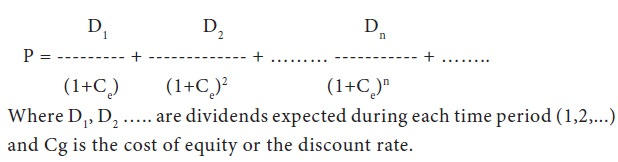The basic problem here is that all the shareholders would have different expectations. This method of calculating the cost of equity would, therefore, need an assumption that all investors have exactly similar dividend expectations. Another problem relates to the determination of future stream of expected dividends. In order to overcome this problem, it has been suggested that we should assume a constant growth rate in dividend. Let D0 be the current dividend, Dn be the dividend in year n, and g be the constant growth rate of dividend. Then,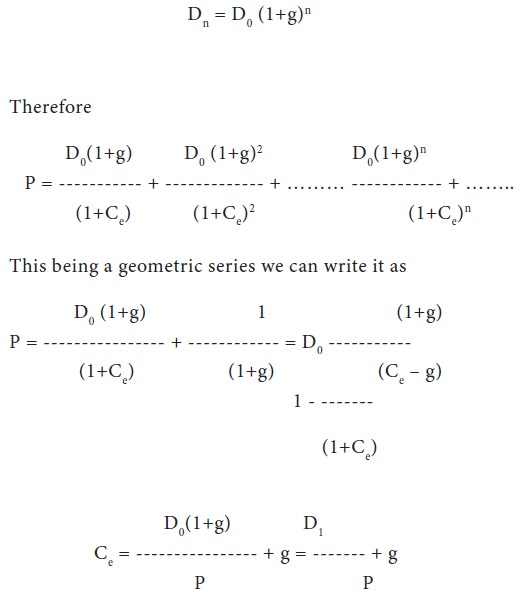Though this method is scientific, one is not sure how to determine the growth rate of dividend (g).

## Method III: Capital Asset Pricing Model (CAPM)

This approach is based on the principle that risk and return of an investment are positively correlated—more risky the investment, higher is the desired returns. This model emphasizes not only the risk differential between equity (or common stock) and government bond but also risk differential among various common stocks.

The P coefficient is used as a risk-index. It measures relative risk among stocks. The beta coefficient may be defined as “the ratio of variability in return on a given stock to variability in return for all stocks,”

The P is calculated by regression analysis, using regression equation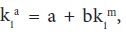where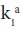is the return on equity of firm a in the ith period and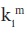is the return on all equity in the market in the ith period. The estimated value of b is known as the beta coefficient.

A beta coefficient of value 1.0 means the stack's return is as volatile or risky as the market's, b > 1 and b < 1 means the stock's return are more volatile and less volatile respectively compared to the return on total stock market portfolio.

In this model the cost of equity capital (re) is: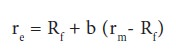Where, Rf refers to risk free rate and r^ to return on market portfolio. Here cost of equity capital is composed of two components: the risk-free rate (Rf ), and (2) the weighted risk component where (rm – Rf ) refers to the overall risk premium, while the risk associated with the firm in question (b) is used as weights.

Tags : Financial Management - Capital Budgeting – A Conceptual Framework
Last 30 days 120 views